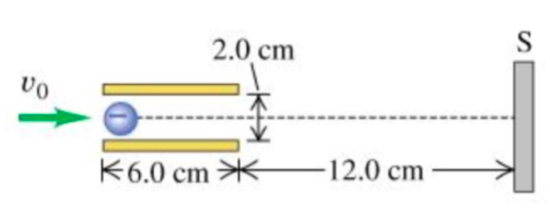# Problem: Cathode-ray tubes (CRTs) are often found in oscilloscopes and computer monitors. In the figure, an electron with an initial speed of 7.00x106 m/s is projected along the axis midway between the deflection plates of a cathode-ray tube. The potential difference between the two plates is 18.0 V and the lower plate is the one at higher potential.A)What is the magnitude of the force on the electron when it is between the plates?B)What is the direction of the force on the electron when it is between the plates?a. Upwardb. DownwardC)What is the magnitude of the acceleration of the electron when acted on by the force in part (a)?D)What is the direction of the acceleration of the electron when acted on by the force in part (a)?a. Upwardb. DownwardE)How far below the axis has the electron moved when it reaches the end of the plates?F)At what angle with the axis is it moving as it leaves the plates?

###### FREE Expert Solution

A)

The magnitude of the force on an electron is expressed as:

$\overline{){\mathbf{F}}{\mathbf{=}}{\mathbf{e}}{\mathbf{E}}}$, where e is the charge of an electron and E is the electric field.

The electric field is found from:

E = ΔV/d where ΔV is the potential difference between the plates and d is the separation of the plates.

96% (458 ratings)###### Problem Details

Cathode-ray tubes (CRTs) are often found in oscilloscopes and computer monitors. In the figure, an electron with an initial speed of 7.00x106 m/s is projected along the axis midway between the deflection plates of a cathode-ray tube. The potential difference between the two plates is 18.0 V and the lower plate is the one at higher potential.A)What is the magnitude of the force on the electron when it is between the plates?

B)What is the direction of the force on the electron when it is between the plates?
a. Upward
b. Downward

C)What is the magnitude of the acceleration of the electron when acted on by the force in part (a)?

D)What is the direction of the acceleration of the electron when acted on by the force in part (a)?
a. Upward
b. Downward

E)How far below the axis has the electron moved when it reaches the end of the plates?

F)At what angle with the axis is it moving as it leaves the plates?